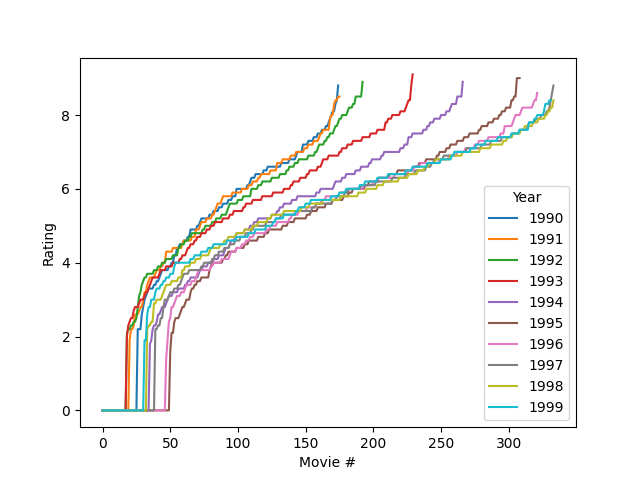# Statistics: Exercises and Solutions

## Correlating activity in the left and right brain

### Exercise

• Read this dataset, which has been adapted from the StudyForrest project. See Exercise 2 from the NumPy tutorial if you don't remember this dataset!
• Get the mean BOLD response over time, separately for the left and the right brain.
• Plot the BOLD response over time for the left and the right brain.
• You will notice that there is a slight drift in the signal, such that the BOLD response globally increases over time. You can remove this with so-called detrending, using `scipy.signal.detrend()`.
• Plot the detrended BOLD response over time for the left and the right brain.
• Determine the correlation between the detrended BOLD response in the left and the right brain.

Statistical caveat: Measures that evolve over time, such as the BOLD response, are not independent. Therefore, statistical tests that assume independent observations are invalid for this kind of data! In this exercise, this means that the p-value for the correlation is not meaningful.

### Solution

```import numpy as np
from matplotlib import pyplot as plt
from scipy.stats import linregress
from scipy.signal import detrend

# Get the average over the left and right brain across all dimensions
# except time
left = a[:34].mean(axis=(0, 1, 2))
right= a[34:].mean(axis=(0, 1, 2))
# Detrend the signals and get the correlation between them
detrended_left = detrend(left)
detrended_right = detrend(right)
slope, intercept, r, p, se = linregress(detrended_left, detrended_right)
print('r = {:.4f}'.format(r))
# Create a nice plot!
xdata = np.arange(0, 90, 10)
plt.subplot(2, 1, 1)
plt.plot(left, label='Left')
plt.plot(right, label='Right')
plt.xticks(xdata, 2 * xdata)
plt.xlabel('Time')
plt.xlim(0, 90)
plt.ylabel('BOLD')
plt.legend()
plt.subplot(2, 1, 2)
plt.plot(detrended_left)
plt.plot(detrended_right)
plt.xticks(xdata, 2 * xdata)
plt.xlabel('Time')
plt.xlim(0, 90)
plt.ylabel('BOLD')
plt.show()
```

Output:

```r = 0.8861
```